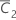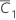# Methods and formulas for the effects table in Equivalence Test for a 2x2 Crossover Design

## Carryover effect

Let C2k and C1k be defined as follows: The carryover effect is then given by:

### SE

The standard error for the carryover effect is given by the following: where Sp is the pooled standard deviation for the carryover effect, which is given by the following:

### Notation

TermDescriptionSample mean using the data C2k, k = 1, ..., n2Sample mean using the data C1k, k = 1, ..., n1
n2Number of participants in sequence 2
n1Number of participants in sequence 1
S2Sample standard deviation of C2k, k = 1, ..., n2
S1Sample standard deviation of C1k, k = 1, ..., n1

## Treatment effect

The treatment effect is given by:

### SE

The standard error for each effect is given by the following: where Sp is the pooled standard deviation which is given by the following:

### Notation

TermDescriptionSample mean for sequence i (for more information, go to Methods and formulas for common concepts used in Equivalence Test for a 2x2 Crossover Design)
niNumber of participants in sequence i
S1Sample standard deviation offor sequence i

## Period effect

The period effect is given by:

### SE

The standard error for each effect is given by the following: where Sp is the pooled standard deviation, which is given by the following:

### Notation

TermDescriptionSample mean for sequence i (for more information, go to Methods and formulas for common concepts used in Equivalence Test for a 2x2 Crossover Design)
niNumber of participants in sequence i
S1Sample standard deviation offor sequence i

## T-value, P-value, and confidence interval for effects

### T-value

The t-value for each effect is given by the following:

### P-value

The p-value for each effect is given by the following: Where t is the observed t-value and T follows a t-distribution with n1 + n2 - 2 degrees of freedom.

### Confidence interval for equivalence

The confidence interval (CI) for equivalence for each effect is given by the following:

### Notation

TermDescription
SEStandard error for the effect (for more information, see the section on each effect)
tα/2Upper α/2 critical value for a t-distribution with n1 + n2 – 2 degrees of freedom
αProbability of rejecting the null hypothesis when the null hypothesis is true (also called the significance level)
niNumber of participants in sequence i
By using this site you agree to the use of cookies for analytics and personalized content.  Read our policy